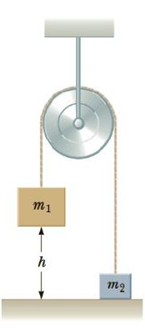Chapter 5, Problem 38P

Chapter
Section
Textbook Problem

Two blocks are connected by a light string that passes over a frictionless pulley as in Figure P5.38. The system is released from rest while m2 is on the floor and m1 is a distance h above the floor. (a) Assuming m1 > m2, Find an expression for the speed of m1 just as it reaches the floor. (b) Taking m1 = 6.5 kg, m2 = 4.2 kg, and h = 3.2 m, evaluate your answer to part (a), and (c) find the speed of each block when m1 has fallen a distance of 1.6 m.Figure P5.38

(a)

To determine
The expression for the speed of m1 just as it reaches the floor.

Explanation

Given Info:

The mass m1 is greater than m2 .

When the mass m1 is falling to the floor, only gravitational force is acting on it. Since, the gravitational force is a conservative force; the total mechanical energy is a constant.

Thus,

KE1,f+KE2,f+(PEg)1,f+(PEg)2,f=KE1,i+KE2,i+(PEg)1,i+(PEg)2,i       (I)

Consider the floor as h=0 level

(b)

To determine
The speed of m1 just as it reaches the floor.

(c)

To determine
The speed of each block when m1 has fallen a distance of 1.6m .

Still sussing out bartleby?

Check out a sample textbook solution.

See a sample solution

The Solution to Your Study Problems

Bartleby provides explanations to thousands of textbook problems written by our experts, many with advanced degrees!

Get Started